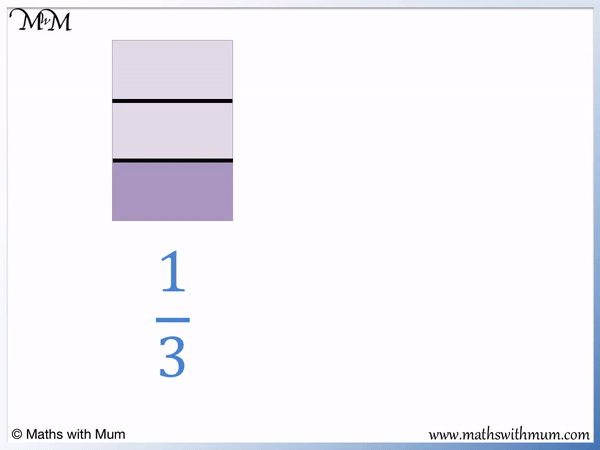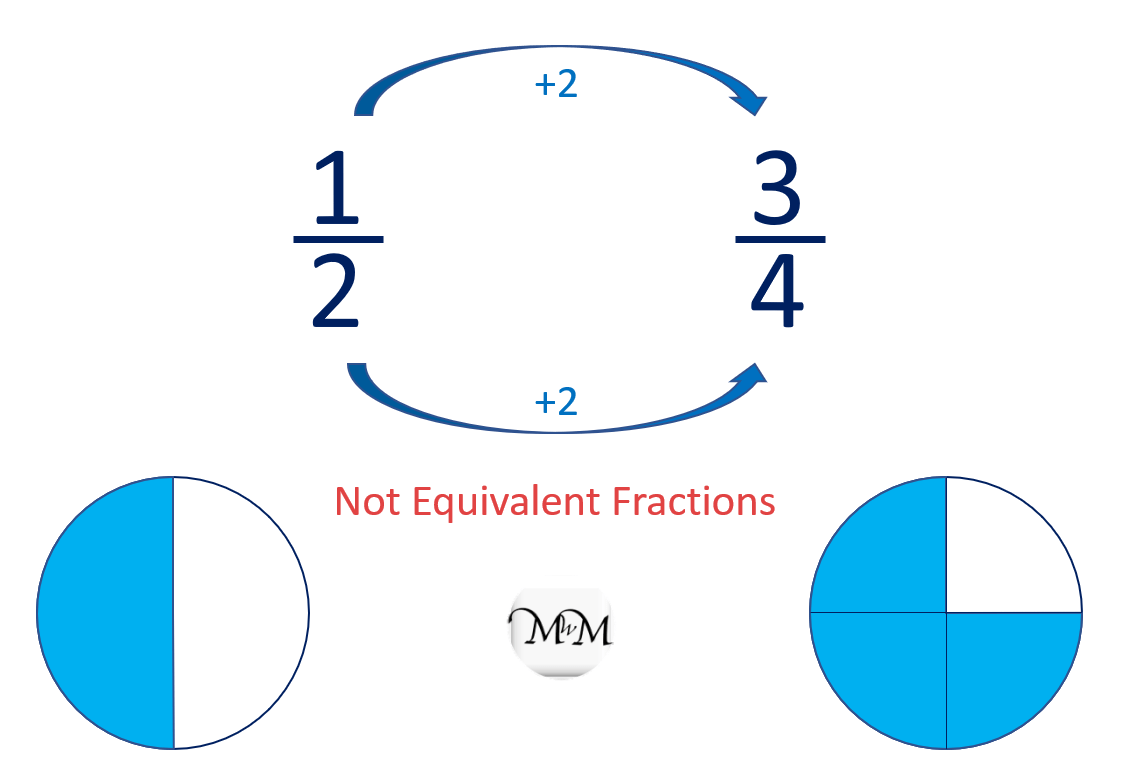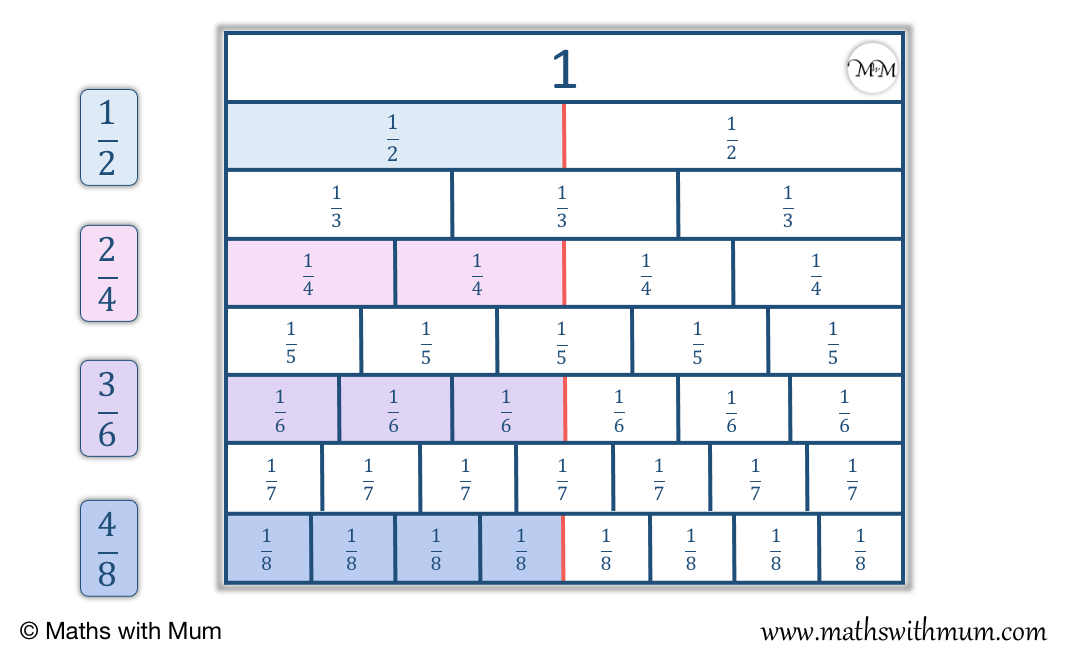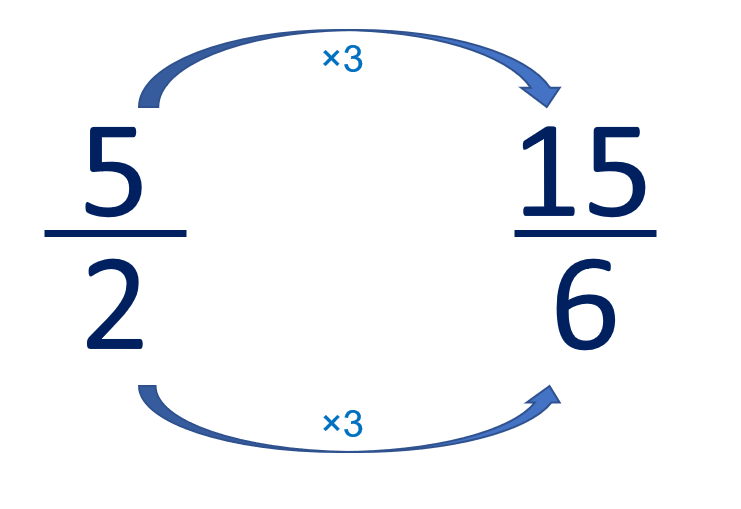# How to Find Equivalent Fractions

How to Find Equivalent Fractions• Equivalent fractions are the same size but are written differently.
• To be equivalent fractions, the and must be multiplied or divided by the same number.
• The two fractions:   1 / 2   and   2 / 4 are equivalent fractions.
• The numerator and denominators were both multiplied by 2.
• We can multipliy the numerator and denominator by 2 again to find another equivalent fraction of   4 / 8  .
• We can multiply both the numerator and denominator of   1 / 2  by 4 to make  4 / 8  .
• We can also divide the numerator and denominator of a fraction to make an equivalent fraction.
• We could choose to multiply both the numerator and denominator by an infinite range of different numbers to make further examples of equivalent fractions.
To make an equivalent fraction, multiply or divide the numerators and denominators by the same number.• To find an equivalent fraction to   1 / 3  , we must multiply both the numerator and denominator by the same number.
• In this example, we chose to multiply by 2.
•   1 / 3  and   2 / 6  are equivalent fractions.
• We can see that they take up the same fraction of the shaded space in the shape shown.# Equivalent Fractions

## What are Equivalent Fractions?

Equivalent fractions are fractions that are the same size but have different numerators and denominators. To be equivalent, the numerator and denominator of a fraction must have been multiplied or divided by exactly the same number to make the new fraction.

For example, in the fraction   2 / 3  , the numerator and denominator can be multiplied by 2 to find the equivalent fraction   4 / 6  .

The numerator of 2 has been doubled to get 4 and the denominator of 3 has been doubled to get 6.

Both fractions are equivalent because   4 / 6 is   2 / 3 .

One third of 6 is 2 and two thirds of 6 is 4.

Therefore 4 is two thirds of 6. This means that 4 / 6 is the same as   2 / 3 .

There are an infinite number of equivalent fractions that a fraction can have. This is because we can choose any number to multiply the numerator and denominator by and there are infinite numbers.

For example, this time instead of multiplying the digits in 2 / 3 by 2, we will multiply by 5.2 multiplied by 5 equals 10 and 3 multiplied by 5 is 15.

We say that fractions that have the same ratio of numerator to denominator are called equivalent.

2 / 3 is equivalent to   10 / 15 .

This also means that 2 / 3 is equivalent to   4 / 6 which is equivalent to 10 / 15 is   2 / 3 .

## How to Find Equivalent Fractions

To find equivalent fractions, follow these steps:

1. Multiply or divide the numerator and denominator by the same number.
2. Only divide if the numerator and denominator remain as whole numbers.

Foe example, 3 / 4 is equivalent to 300 / 400 .The numerator and the denominator have been multiplied by the same amount, so they are equivalent.

The numerator and denominator have both been multiplied by 100.

3 × 100 = 300 and 4 × 100 = 400.

When teaching equivalent fractions, it is important to state that we only multiply and divide and never add or subtract. It is a common mistake for children to add or subtract because they are often more familiar with these processes than they are with multiplication and division.

We can see this common mistake easily in the following diagram. When teaching equivalent fractions, it can help to show this common mistake using a diagram.

We can see that the first fraction is 1 / 2 but by adding 2 to both the numerator and denominator, we get to the fraction 3 / 4 .We can see that 3 / 4 is clearly a larger fraction than 1 / 2 and so, the fractions are not equivalent.

## Why Does the Rule for Equivalent Fractions Work?

The rule for equivalent fractions works because the numerator and the denominator are both multiplied or divided by the same number. Increasing the numerator increases the size of the fraction and increasing the denominator decreases the size of the fraction.

If the numerator and denominator are multiplied or divided by the same amount, the fraction has increased and decreased by the same amount and so, it has not changed its overall size.

The denominator of a fraction tells us how many parts it is split up into in total.

The numerator of a fraction tells us how many parts we have.

If we double the number of parts there are in total and also double the number of parts we have, the proportion of parts we have has not changed.

For example, in the fraction 1 / 2 , we have 1 part shaded out of 2.If we double the number of parts we have in total, then each part is twice as small. We now need two parts to have half of the shape.

1 / 2 is equivalent to 2 / 4 . This is because 2 is half of 4.

If we double the number of parts again, each part becomes even smaller. Each part is now four times smaller than the half parts that we had originally.

1 / 2 is equivalent to 4 / 8 . This means that 4 is half of 8.

All of these fractions are equivalent because they take up the same space. The diagrams show that they are the same amount of the total shape shaded.

## Equivalent Fractions Chart

An equivalent fractions chart is a visual way to show fractions that are equivalent to each other. Fractions that are the same size are shown highlighted on an equivalent fraction chart.

Here is an equivalent fraction chart showing fractions equivalent to 1 / 2.There are infinite fraction that are equivalent to one half, such as 2 / 4 and 3 / 6 .

Here is an equivalent fraction chart showing fractions equivalent to 1 / 3 .There are infinite fractions that are equivalent to one third, such as 2 / 6 or 3 / 9 .

Fraction charts are also known as fraction walls and can be a very useful method of teaching the concept of equivalent fractions.

An activity for children can be to look for and identify equivalent fractions using a fraction wall.

## Improper Equivalent Fractions

Two Improper fractions can be equivalent too. To find equivalent improper fractions, multiply or divide the numerator and the denominator by the same amount.

For example here is the improper fraction 5 / 2 .

We can multiply the numerator and denominator by any amount to make an equivalent fraction as long as this number is the same.In this example, we have multiplied the numerator and denominator both by 3.

5 / 2 is equivalent to 15 / 6 .

## Where are Equivalent Fractions Used in Real Life?

Here are some examples of using equivalent fractions in real life:

• Finding amounts of ingredients to use in cooking.
• Sharing out food that is cut into slices.
• Working out Percentages.

For example, you are following a recipe to make a cake that requires half a cup of water. If the cup you are measuring it in only has measurements in quarters, then you can use equivalent fractions to know that 2 quarters are the same as one half.

If you are sharing a 12 slice pizza between 2 people, then you will know that 1 / 2 is equivalent to 6 / 12 and so, you get 6 slices each.

Percentages are found in real life in test scores, computer downloads and many other places. Percentages are fractions out of 100. To convert to a percentage, we need to use equivalent fractions to find a fraction out of 100.

For example, if you scored 12 out of 20 in a test and wanted to know what percentage this was, you would use equivalent fractions.

Multiplying the numerator and denominator by 5 would convert 12 / 20 to 60 / 100 and so, you would have scored 60%. Equivalent fractions are used in real life whenever we need to convert to a percentage.Now try our lesson on Equivalent Fractions: Missing Numerator or Denominator where we learn how to find a missing numerator or denominator in an equivalent fraction.error: Content is protected !!